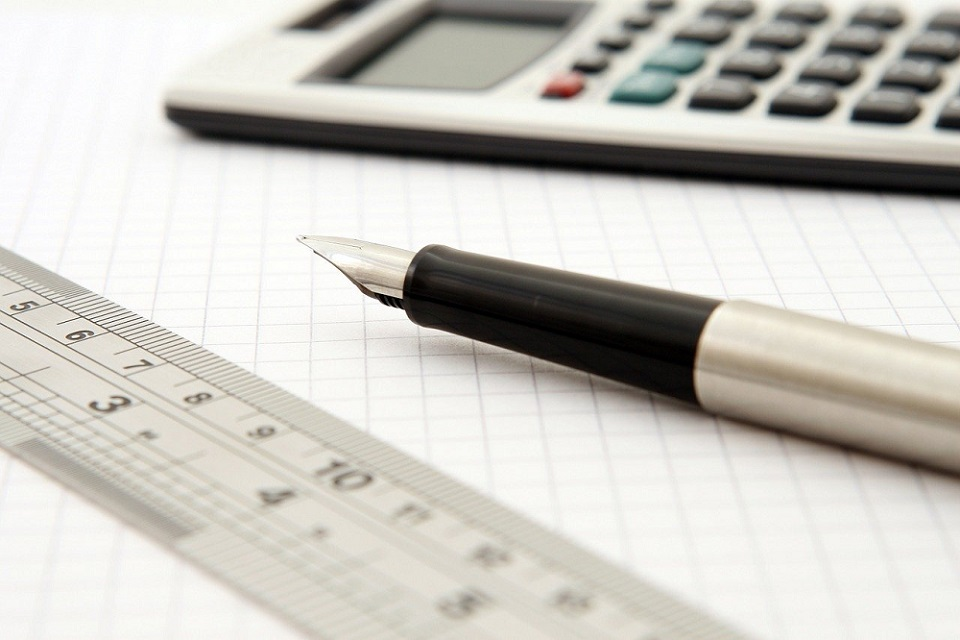## Breaking News

### Encouraging Houses: The Effect of Lambeth Electricians on Local Authority# 6 Simple and Easy Ways to do Standard Form

## Standard form Representation of Numbers

The standard form has numerous uses in mathematics. Therefore, it is essential to know what is the standard form and how we can change numbers into it when required. Writing a complex number into a simple and easy to read format is known as the standard form. There are some simple and easy ways discussed below to do it. A standard notation calculator is another way to get the desired form without wrenching nerve calculations.

### Standard Form Numbers

This method consists of the following steps:

Observe the written word and change it into the number. For example, if you have a number “seven thousand four hundred thirty-eight,” you will need to write it in numerical form.

We will do a step by step process:

• Seven thousand = 7000
• Four hundred = 400
• Thirty = 30
• Eight = 8
• Now we will add them: 7000 + 400 + 30 + 8 = 7438
• In the results, we will have our final answer, that is: “seven thousand four hundred thirty-eight” is 7438.

### Decimal Number’s Standard Form

This method is used to handle very small or very large numbers. It is also recognized as scientific notation.

• Assume any number such as 4.29000000000.
• Locate the decimal and count the numbers after it. In this example, there are 11 digits.
• Write it down with the multiple of 10, and it will be 4.29 * 1011It is the final standard form.

This method is highly recommended to shift from scientific notation to standard form. However, for this purpose, you can write in a scientific notation calculator as well to get the desired output.

### Variable Equation Method

It is the way to deal with the equation. Let’s assume we have A: x5 + 9 = -9 + 9

• We have to solve both sides of the given equation to have a standard form. Such as we will have A: x5 + 9 = 0 after solving it.

### Polynomial Method

In this form, any kind of equation will be present. For instance, we have x + 2×3 – 4×4 + 7×2 + x5 = 10.

• Move all the numbers on one side of the equation. In present case we will move 10 and as a result: 8x + 2×3 – 4×4 + 7×2 + x5 – 10 = 0
• Rearrange the equation from higher to lower degree to have the required outcomes; x5 – 4×4 + 2×3 + 7×2 + 8x – 10 = 0

### Linear Equation Method

This method is preferred while dealing with linear equations such as Ax + By = C

• Change the above equation. 7X + 4Y = 2 will be converted into y/2 = 7x – 4 after shifting variables.
• Now remove fractions to get 3y = 14x – 8.
• The constant that is -8 will be isolated as 3y – 14x = -8
• Rearrange the variables to make the constant or lead coefficient positive to get 14x – 3y = 8.